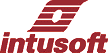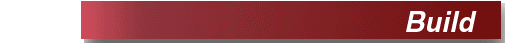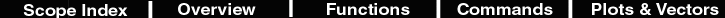Apply han 10% taper to current waveform
 Description Build and apply han10 to current waveform Makes a new vector which starts and ends at the average of the current vector using a cosine taper for the first and last 5% of the current waveform. An error is sent to the output window if there is no scale. When a waveform is transformed into frequency domain using a discrete Fourier transform it is treated as though it is periodic. Artificial high frequency components appear in the frequency domain if the waveform doesn't begin and end with the same value. These windows force the value to zero at the endpoints if they are applied (multiplied) directly. For sine waves this works out reasonably well, however, cosine waves will introduce a large transient. To remove this effect, the initial and final values are offset so they are nearly zero before applying the window. Then the offset is restored after applying the window. Using windowing functions will smear out an otherwise sharp spectral line. Data from simulation can generally be controlled to begin and end with the simulation span so that application of windowing functions is not necessary. Hot Key none Script if length(default) > 1 set units = rad homecursors delx_ = getcursorx(1) - getcursorx(0) taper_ = .05 alias previous = current x1_ = taper_ * delx_ + default set units = rad homecursors delx_ = getcursorx(1) - getcursorx(0) taper_ = .05 x1_ = taper_ * delx_ + default x2_ = (1 - taper_) * delx_ + default pi = 4*atan(1) han10 = unitvec(length(default))*(1+taper_) n = 0 while default[n] < x1_ han10[n] =.5* han10[n]*(1 - cos(pi*default[n]/x1_) ) n = n+1 end n = length(default)-1 while default[n] > x2_ han10[n] = .5*han10[n]*(1+ cos(pi*(default[n]-x2_)/x1_) ) n = n - 1 end set units = deg han10 = han10 / average(han10) vo_ = (current + current[length(current)-1])/2 result =vo_ + han10*(current - vo_) plotf result han10(%s) previous else print "This plot contains no scale" end Example Open scope and paste this script into the Command Window. Then press to plot a sine wave. Select "Build and apply han10 to current waveform" from the Build menu to view the result. set units = rad pi =4*atan(1) numpoints = 1000 time = (vector(numpoints)/(numpoints-1)*1m) newplot sineplot time setplot sineplot y = sin(2*pi*time*5.25k) plot y setcursor 1 500u set units = deg print 2/pi

Apply han 20% taper to current waveform
 Description Build and apply han20 to current waveform. see han10 for details Hot Key none Script set units = rad homecursors delx_ = getcursorx(1) - getcursorx(0) taper_ = .1 see han10 for the remaining script Example see han10

Apply han 100% taper to current waveform
 Description Build and apply han100 to current waveform. see han10 for details Hot Key none Script set units = rad homecursors pi = 4*atan(1) previous = current han100 = 1 - cos(2*pi*default/(getcursorx(1) - getcursorx(0))) set units = deg vo_ = (current + current[length(current)-1])/2 result =vo_ + han100*(current - vo_) plotf result han100(%s) previous Example see han10

Black Body
 Description Generate and plot black-body radiation curves in the visible spectrum for 3200, 6000 and 9000 deg K This script illustrates many ICL features. First it loads rule.plt; the defining plot for dimensional (units) analysis. This is a user extensible file that defines the relationship between basic units; for example watt = volt*amp. It is a special plot in which units are not evaluated. The script then copies the units into the constants plot so that they can be used to define units for the various constants we will use for this problem. Constants are then defined along with their units. We set the angle computation to radians and reset it at the end to degrees. The function eb(t,u), is the black body radiation function taken from a text book. The frequency, u, will be passed into the function as a vector. That forces the left hand side of the equation to also be a vector of the same length. What that means to you, the user, is that the expression evaluation will automatically loop through each wavelength using an efficient C language coded loop that runs thousands of times faster than an equivalent interpretive language like Visual Basic. The vector function is built into the ICL language and creates a vector from 0 to the magnitude of its argument, with the number of elements equal to the argument. "wavelength" is then the x-axis scale for the blackbody plot, running from 400 nanometers to (400+380) nanometers; the visible specrum of light. Newplot and setplot make the plot; copying wavelength over as the default vector. The keyword "default" can then refer to the wavelength. "c/default" is evaluated to be a vector and its vector property causes the function eb() to be evaluated as a vector for each of the wavelengths. Its then a simple matter to plot each curve, normalized to its own mean. Dimensional analysis proceeds as you go so that dimensional errors ill wind up with strange or unknown units. Hot Key none Script load rule.plt nv = nextvector(null) while nv != null constants.nv = nv nv = nextvector(nv) end setplot constants h = 6.62607e-034 * joule * time k = 1.38e-23 * joule / kelvin c = 300meg * length / time e = exp(1) set units = rad function eb(t,u) ((2 * pi * h * u^3)/(c^2 * (e^((h * u) / (k *t)) - 1))) print h k c pi e wavelength = length*(vector(100)/99*400n+380n) newplot blackbody wavelength setplot blackbody w_3200 = eb(3200*kelvin,c/default) w_3200 = w_3200/mean(w_3200) plot w_3200 w_6000 = eb(6000*kelvin,c/default) w_6000 = w_6000/mean(w_6000) plot w_6000 w_9000 = eb(9000*kelvin,c/default) w_9000 = w_9000/mean(w_9000) plot w_9000 set units = deg Example This script produces a standalone result.

Cosine Window
 Description Make a plot called cosw which is cos(.5*pi*x/xmax) This window is commonly applied to frequency domaing data to provide a low pass filter. It can be raised to a power to reduce the pass band and sharpen the filter. Hot Key none Script set units = rad homecursors pi = 4*atan(1) cosw = cos(.5*pi*default/(getcursorx(1) - getcursorx(0))) set units = deg plot cosw Example This script produces a standalone result.

hanning 10 percent taper
 Description Makes a plot called han10 which is cosine tapered 5% on each end and has an average value of 1. The taper percentage reverts to the percent of the original waveform that is altered. Hot Key none Script if length(default) > 1 set units = rad homecursors delx_ = getcursorx(1) - getcursorx(0) taper_ = .05 x1_ = taper_ * delx_ + default set units = rad homecursors delx_ = getcursorx(1) - getcursorx(0) taper_ = .05 x1_ = taper_ * delx_ + default x2_ = (1 - taper_) * delx_ + default pi = 4*atan(1) han10 = unitvec(length(default))*(1+taper_) gate = ~pulse(x2_) gate = gate + pulse(x1_) han10c = ~gate*han10 han10 = han10c + .5* (1 - cos(pi*default/x1_))*gate han10 = han10 / average(han10) plot han10 else print "This plot contains no scale" end Example This script produces a standalone result.

hanning 20 percent taper
 Description Makes a plot called han20 which is cosine tapered 10% on each end and has an average value of 1. See above Hot Key none Script if length(default) > 1 set units = rad homecursors delx_ = getcursorx(1) - getcursorx(0) taper_ = .1 x1_ = taper_ * delx_ + default set units = rad homecursors delx_ = getcursorx(1) - getcursorx(0) x1_ = taper_ * delx_ + default x2_ = (1 - taper_) * delx_ + default pi = 4*atan(1) han20 = unitvec(length(default))*(1+taper_) n = 0 while default[n] < x1_ han20[n] =.5* han20[n]*(1 - cos(pi*default[n]/x1_) ) n = n+1 end n = length(default)-1 while default[n] > x2_ han20[n] = .5*han20[n]*(1+ cos(pi*(default[n]-x2_)/x1_) ) n = n - 1 end han20 = han20 / average(han20) set units = deg plot han20 else print "This plot contains no scale" end Example This script produces a standalone result.

hanning 100 percent taper
 Description Makes a plot called han100 which is cosine tapered 50% on each end and has an average value of 1. See above Hot Key none Script if isdef(default) = 1 set units = rad homecursors pi = 4*atan(1) han100 = 1 - cos(2*pi*default/(getcursorx(1) - getcursorx(0))) set units = deg plot han100 else print "This plot contains no scale" end Example This script produces a standalone result.